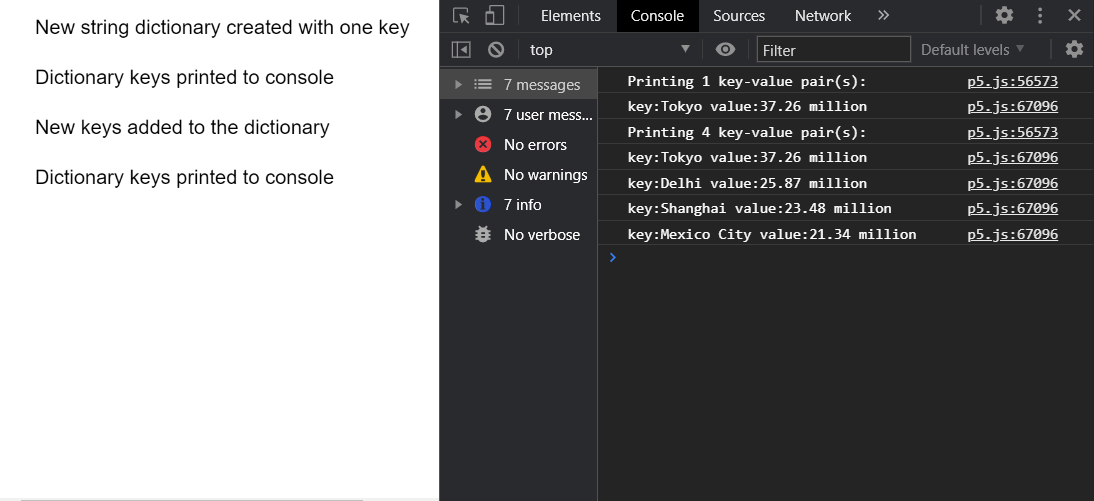Open in App
Not now

# p5.js TypedDict print() Method

• Last Updated : 27 Nov, 2020

The print() method of p5.TypedDict in p5.js is used to print out all the key-value pairs currently present in the dictionary to the console. A key-value pair is a set of two values that are mapped to each other. These values can be accessed by querying this dictionary using the key portion of the pair. A typed dictionary can store multiple key-value pairs that can be accessed using the methods of the dictionary.

Syntax:

```print()
```

Parameters: This method does not accept any parameters.

The example below illustrates the print() method in p5.js:

Example:

## Javascript

 `function` `setup() {``  ``createCanvas(550, 300);``  ``textSize(16);`` ` `  ``let stringDict = ``      ``createStringDict(``"Tokyo"``, ``"37.26 million"``);``  ``text(``"New string dictionary created "` `+``       ``"with one key"``, 20, 20);`` ` `  ``// Getting the current size of the dictionary``  ``let currSize = stringDict.size();``  ``print(``"Printing "` `+ currSize +``        ``" key-value pair(s):"``);`` ` `  ``// Printing all the values to console``  ``stringDict.print();``  ``text(``"Dictionary keys printed to console"``,``       ``20, 60);`` ` `  ``let tmpObj = {``    ``"Delhi"``: ``"25.87 million"``,``    ``"Shanghai"``: ``"23.48 million"``,``    ``"Mexico City"``: ``"21.34 million"``  ``};`` ` `  ``// Add the given key to the dictionary``  ``// specifying the key and value as an object``  ``stringDict.create(tmpObj);``  ``text(``"New keys added to the dictionary"``,``       ``20, 100);`` ` `  ``// Getting the current size of the dictionary``  ``currSize = stringDict.size();``  ``print(``"Printing "` `+ currSize +``        ``" key-value pair(s):"``);`` ` `  ``// Printing all the values to console``  ``stringDict.print();``  ``text(``"Dictionary keys printed to console"``,``       ``20, 140);``}`

Output:Online editor: https://editor.p5js.org/
Environment Setup: https://www.geeksforgeeks.org/p5-js-soundfile-object-installation-and-methods/
Reference: https://p5js.org/reference/#/p5.TypedDict/print

My Personal Notes arrow_drop_up